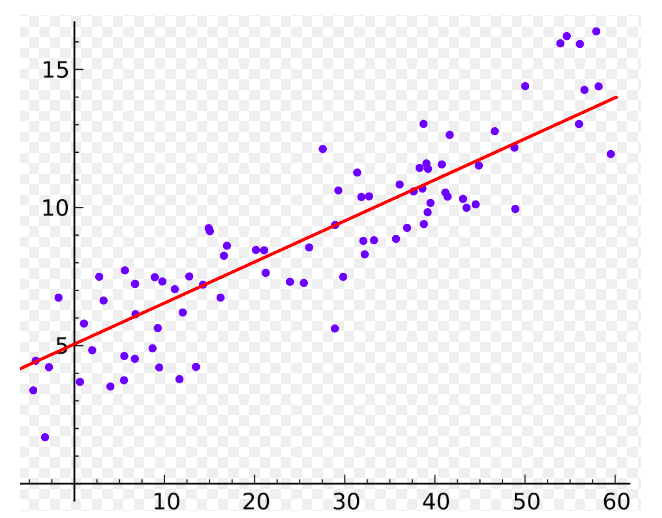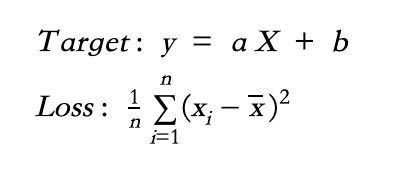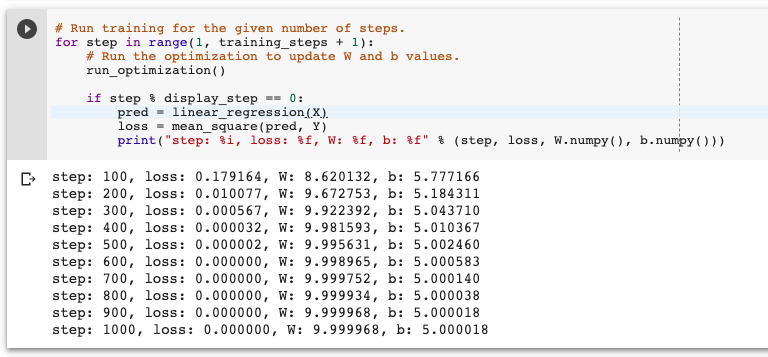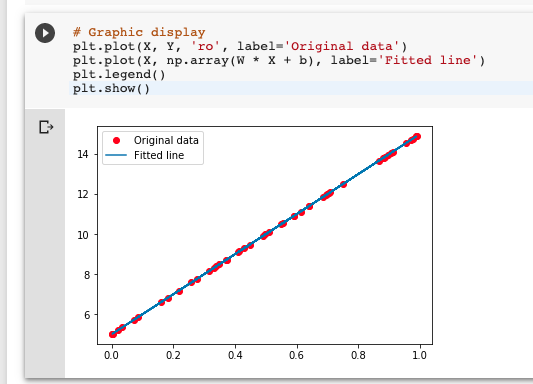#DAY 6
0

## [Day-6] Tensorflow Linear regressionSource

Simple Linear regression

Simple linear regression就是在二維平面上找出一個，能代表資料的一條直線，接下來我們會用TF來簡單跑一個simple linear regression。

``````#Create dataset
X = np.random.rand(n_samples).astype(np.float32)
Y = X * 10 + 5
W = tf.Variable(tf.random.normal())
b = tf.Variable(tf.zeros())
````````````#Define LR and Loss function (MSE)
def linear_regression(x):
return W * x + b

def mean_square(y_pred, y_true):
return tf.reduce_sum(tf.pow(y_pred-y_true, 2)) / (2 * n_samples)

optimizer = tf.optimizers.SGD(learning_rate)
``````

``````def run_optimization():
# Wrap computation inside a GradientTape for automatic differentiation.
pred = linear_regression(X)
loss = mean_square(pred, Y)

# Update W and b following gradients.
``````

``````for step in range(1, training_steps + 1):
# Run the optimization to update W and b values.
run_optimization()
if step % display_step == 0:
pred = linear_regression(X)
loss = mean_square(pred, Y)
print("step: %i, loss: %f, W: %f, b: %f" % (step, loss, W.numpy(), b.numpy()))
``````Source

TF2_LR

TF1.X_LR

### 2 則留言

0

Dan iT邦新手 5 級 ‧ 2019-09-23 16:29:06 檢舉

0

`if step % display_step == 0:`這段代表什麼意思呢？